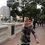# Geometry Set

The problems are in no particular order. Please reshare the note if you like what you see, feel free to share comments or ideas and enjoy.

1. Let $$P$$ and $$Q$$ be points on the side $$AB$$ of the triangle $$ABC$$ (with $$P$$ between $$A$$ and $$Q$$) such that $$\angle{ACP}=\angle{PCQ}=\angle{QCB}$$, and let $$AD$$ be the angle bisector of $$\angle{BAC}$$ with $$D$$ on $$BC$$. Line $$AD$$ meets lines $$CP$$ and $$CQ$$ at $$M$$ and $$N$$ respectively. Given that $$PN=CD$$ and $$3\angle{BAC}=2\angle{BCA}$$, prove that triangles $$CQD$$ and $$QNB$$ have the same area. (Belarus 1999)

2. Let $ABC$ be a triangle and let $\omega$ be its incircle. Let $D_1$ and $E_1$ be the tangency points of $\omega$ with sides $BC$ and $AC$, respectively. Let $D_2$ and $E_2$ be points on sides $BC$ and $AC$, respectively, such that $CD_2=BD_1$ and $CE_2=AE_1$, and let $P$ be the intersection point of segments $AD_2$ and $BE_2$. Circle $\omega$ intersects segment $AD_2$ in two points, being the closest one to $A$ denoted by $Q$. Prove that $AQ=D_2P$.

3. Points $A, B, C, D$ are four consecutive vertices of a regular polygon such that $\dfrac{1}{AB}=\dfrac{1}{AC}+\dfrac{1}{AD}$. How many sides does the polygon have?Note by José Marín Guzmán
6 years, 6 months ago

This discussion board is a place to discuss our Daily Challenges and the math and science related to those challenges. Explanations are more than just a solution — they should explain the steps and thinking strategies that you used to obtain the solution. Comments should further the discussion of math and science.

When posting on Brilliant:

• Use the emojis to react to an explanation, whether you're congratulating a job well done , or just really confused .
• Ask specific questions about the challenge or the steps in somebody's explanation. Well-posed questions can add a lot to the discussion, but posting "I don't understand!" doesn't help anyone.
• Try to contribute something new to the discussion, whether it is an extension, generalization or other idea related to the challenge.
• Stay on topic — we're all here to learn more about math and science, not to hear about your favorite get-rich-quick scheme or current world events.

MarkdownAppears as
*italics* or _italics_ italics
**bold** or __bold__ bold
- bulleted- list
• bulleted
• list
1. numbered2. list
1. numbered
2. list
Note: you must add a full line of space before and after lists for them to show up correctly
paragraph 1paragraph 2

paragraph 1

paragraph 2

[example link](https://brilliant.org)example link
> This is a quote
This is a quote
    # I indented these lines
# 4 spaces, and now they show
# up as a code block.

print "hello world"
# I indented these lines
# 4 spaces, and now they show
# up as a code block.

print "hello world"
MathAppears as
Remember to wrap math in $$ ... $$ or $ ... $ to ensure proper formatting.
2 \times 3 $2 \times 3$
2^{34} $2^{34}$
a_{i-1} $a_{i-1}$
\frac{2}{3} $\frac{2}{3}$
\sqrt{2} $\sqrt{2}$
\sum_{i=1}^3 $\sum_{i=1}^3$
\sin \theta $\sin \theta$
\boxed{123} $\boxed{123}$

## Comments

Sort by:

Top Newest

3)The question number 3 is easy to solve with complex numbers.But also found a geometric solution.

Let the polygon be $n$- sided.

Suppose the vertex after $D$ be $E$.Hence the $5$ consecutive vertices are $A,B,C,D,E$ ( arranged anticlockwise from A to E,say)

Firstly,$\frac{1}{AB}=\frac{1}{AC}+\frac{1}{AD}$ gives

$AC.AD=AB.AD+AB.AC$...$(1)$

One can easily show that quadrilateral $ACDE$ is cyclic.

So,by Ptolemy's theorem, $AE.CD+AC.ED=AD.EC$...$(2)$

Also,since the polygon is regular,we have $AB=CD=ED$ and $EC=AC$.

So,from $(1)$, $AB.AD+AB.AC=AC.AD=CE.AD=AE.CD+AC.ED=AE.AB+AC.AB$

Hence,$AD=AE$.

Since,these two diagonals are equal,number of vertices between $A$ and $E$(moving clockwise) equals the number of vertices between $A$ and $D$(moving anti-clockwise).

Hence,$n-5=2$ or $n=7$.

- 6 years, 6 months ago

Log in to reply

×

Problem Loading...

Note Loading...

Set Loading...